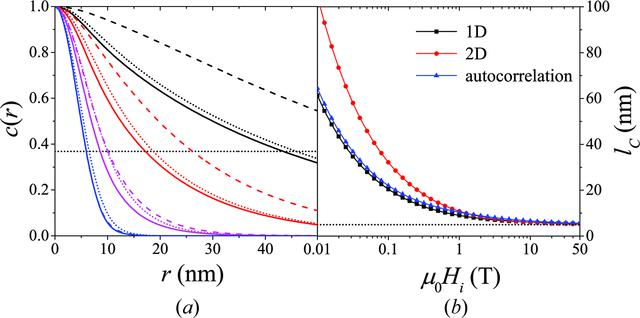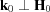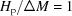disable zoom     view article Figure 13 (a) Comparison between the one-dimensional [equation (36); solid lines] and the averaged two-dimensional [equation (41); dashed lines] correlation functions of the spin-misalignment SANS cross section and the autocorrelation function of the spin misalignment (dotted lines, see Appendix A) [;; S(q) = 1]. c(r) at selected Hi; values of Hi (in T) increasing from top to bottom: 0.02, 0.15, 1.2, 11. Dotted horizontal line: c(r) = exp(-1). (b) Corresponding lC(Hi) (log–linear scale) (solid lines are guides to the eyes). Dotted horizontal line: lC = R = 5 nm.JOURNAL OFAPPLIEDCRYSTALLOGRAPHY
ISSN: 1600-5767
Volume 48| Part 5| October 2015| Pages 1437-1450